# Hyperbola

Hyperbola is defined as an open curve having two branches which are mirror images to each other. It is two curves that are like infinite bows. Here, we will be studying the hyperbola equation, focii, eccentricity, directrix, latus rectum and characteristics of such curves.

## What is Hyperbola?

A hyperbola is a locus of points in such a way that the distance to each focus is a constant greater than one. In other words, the locus of a point moving in a plane in such a way that the ratio of its distance from a fixed point (focus) to that from a fixed line (directrix) is a constant greater than 1.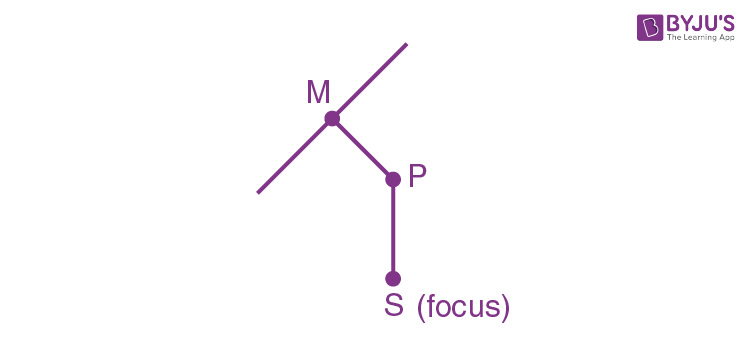[Note: The point (focus) does not lie on the line (directrix)]

(PS/PM) = e > 1 (eccentricity)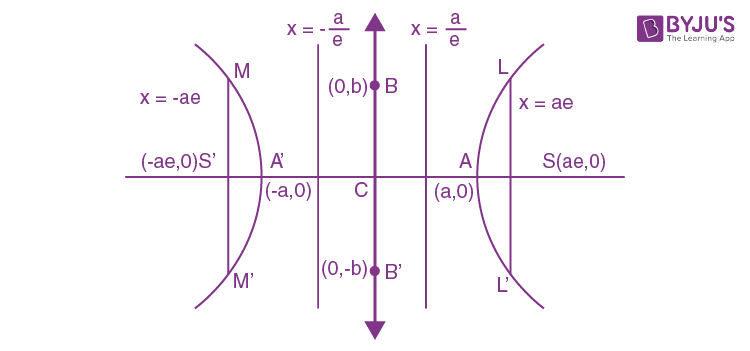### Standard Equation of Hyperbola

The equation of the hyperbola is simplest when the centre of the hyperbola is at the origin and the foci are either on the x-axis or on the y-axis.  The standard equation of a hyperbola is given as:

[(x2 / a2) – (y2 / b2)] = 1

where , b2 = a2 (e2  – 1)

### Important Terms and Formulas of Hyperbola

There are certain terms related to a hyperbola which needs to be thoroughly understood to be able to get confident with this concept. Some of the most important terms related to hyperbola are:

• Eccentricity (e): e2 = 1 + (b2 / a2) = 1 + [(conjugate axis)2 / (transverse axis)2]
• Focii: S = (ae, 0) & S′ = (−ae, 0)
• Directrix: x=(a/e), x = (−a / e)
• Transverse axis:

The live segment A’A of length 2a in which the focii S’ and S both lie is called the transverse axis of the hyperbola.

• Conjugate axis:

The line segment B’B of length 2b between the 2 points B’ = (0, -b) & B = (0, b) is called the conjugate axis of the hyperbola.

• Principal axes:

The transverse axis & conjugate axis.

• Vertices:

A = (a, 0) & A’ = (-a, 0)

• Focal chord:

A chord which passes through a focus is called a focal chord.

• Double ordinate:

Chord perpendicular to the transverse axis is called a double ordinate.

• Latus Rectum:

Focal chord ⊥r to the transverse axis is called latus rectum.

Its length = (2b2 / a) = [(conjugate)2 / transverse] = 2a (e2 − 1)

The difference in focal distances is a constant

i.e. |PS−PS′| = 2a

Length of latus rectum = 2 e × (distance of focus from the corresponding directrix)

End points of L.R : (± ae, ± b2 / a)

Centre:

The point which bisects every chord of the conic, drawn through it, is called the centre of the conic.

C: (0, 0) is the centre of [(x2 / a2) – (y2 / b2)] = 1

Note:

You will notice that the results for ellipse are also applicable for a hyperbola. You need to replace b2  by (−b2)

Recommended Video### Practice Problems on Hyperbola

Example 1:

Find the equation of the hyperbola whose directrix is 2x + y = 1, focus (1, 2) and eccentricity √3.

Solution:

Let P(x, y) be any point on the hyperbola.

Draw PM perpendicular from P on the directrix,

Then by definition SP=ePM.

⇒ (SP)2 = e2 (PM)2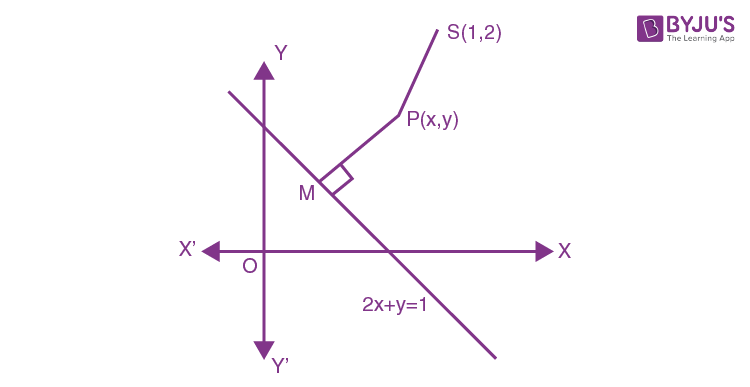⇒ (x − 1)2 + (y − 2)2 = 3{(2x + y – 1) / √(4+1)}2

⇒ 5 (x2 + y2 − 2x − 4y + 5)

= 3 (4x2 + y2 + 1 + 4xy − 2y − 4x)

⇒ 7x2 − 2y2 + 12xy − 2x + 14y – 22 = 0

Which is the required hyperbola.

Example 2:

Find the eccentricity of the hyperbola whose latus rectum is half of its transverse axis.

Solution:

Let the equation of hyperbola be [(x2 / a2) – (y2 / b2)] = 1

Then transverse axis = 2a and latus – rectum = (2b2 / a)

According to question (2b2 / a) = (1/2) × 2a

⇒ 2b2 = a2 (Since, b2 = a2 (e2 − 1))

⇒ 2a2 (e2 − 1) = a2

⇒ 2e2 – 2 = 1

⇒ e2 = (3 / 2)

∴ e = √(3/2)

Hence the required eccentricity is √(3/2)

## What is Conjugate Hyperbola?

2 hyperbolas such that transverse & conjugate axes of one hyperbola are respectively the conjugate & transverse axis of the other are called conjugate hyperbola of each other.

(x2 / a2) – (y2 /b2) = 1 & (−x2 / a2) + (y2 / b2) = 1 are conjugate hyperbolas of each other.

(y2 / b2) – (x2 / a2) = 1

a2 = b2 (e2 − 1)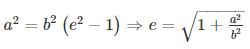Vertices: Vertices: (0,±b) and L.R. = (2a2 / b)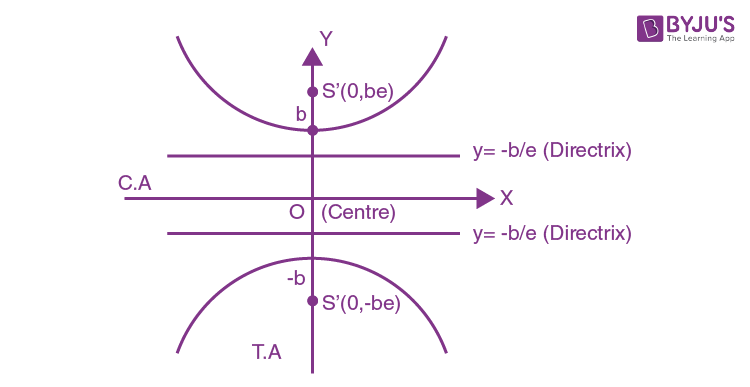### Some Important Conclusions on Conjugate Hyperbola

(a) If are eccentricities of the hyperbola & its conjugate, the

(1 / e12) + (1 / e22) = 1

(b) The foci of a hyperbola & its conjugate are concyclic & form the vertices of a square.

(c) 2 hyperbolas are similar if they have the same eccentricities.

(d) 2 similar hyperbolas are equal if they have the same latus rectum.

Example 3:

Find the lengths of transverse axis and conjugate axis, eccentricity, the co-ordinates of foci, vertices, length of the latus-rectum and equations of the directrices of the following hyperbola 16x2 − 9y2 = −144.

Solution:

The equation 16x2 − 9y2 = −144 can be written as (x2 / 9) – (y2 / 16) = −1

This is of the form (x2 / a2) – (y2 / b2) = −1

∴ a2 = 9, b2 = 16

⇒ a=3, b=4

Length of transverse axis: The length of transverse axis = 2b = 8

Length of conjugate axis: The length of conjugate axis = 2a = 6

Eccentricity: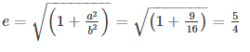Foci: The co-ordinates of the foci are (0, ± be) i.e., (0, ± 5)

Vertices: The co-ordinates of the vertices are (0, ± b) i.e. (0, ± 4)

Lengths of latus-rectum: The length of latus-rectum = (2a2 / b) = [2(3)2] / 4 = 9/2

Equation of directrices: The equation of directrices are

y = ± (b/e) ⇒y = ± [4 / (5/4)] ⇒ y ± (16 / 5)

### Auxiliary Circles of the Hyperbola

A circle drawn with centre C & transverse axis as a diameter is called the auxiliary circle of the hyperbola. The auxilary circle of hyperbola equation is given as:

Equation of the auxiliary circle is x2 + y2 = a2,

Note from the following figure that P & Q are called the “corresponding points” of the hyperbola & the auxiliary circle.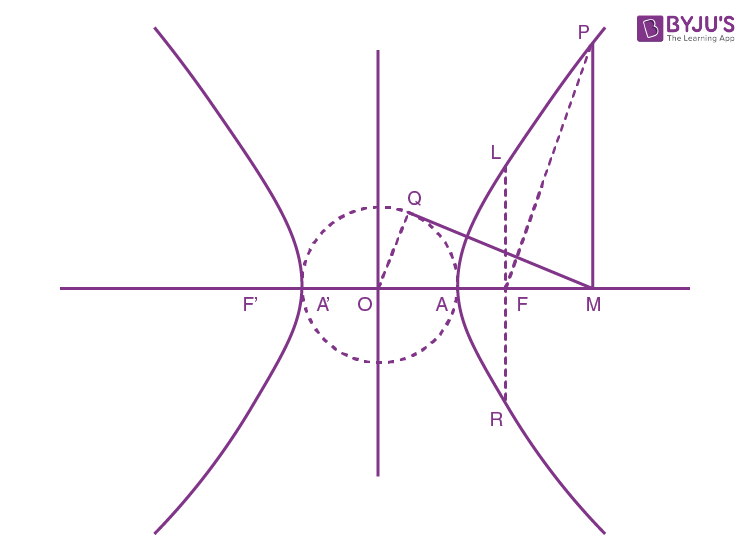Parametric Representation:

x = a sec θ and y = b tan θ

i.e. If (a sec θ, b tan θ) is on the hyperbola, then Q : (a cos θ, a sin θ) lies on the auxiliary circle. The equation of chord joining 2 points P (α) and Q (f) is given by: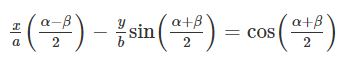The position of a point P

S1 = x12/a2 + y2/b2 = 1 is positive, zero or negative accordingly as (x1 ,y1) lies inside, on or outside is positive, zero or negative accordingly as (x1 ,y1) lies inside, on or outside.

Example 4: Find the position of the point (5, -4) relative to the hyperbola 9x2 – y2 = 1.

Solution:

Since 9(5)2 – (-4)2 – 1 = 225 – 16 – 1 = 208 > 0,

So, the point (5, -4) lies inside the hyperbola 9x2 – y2 = 1.

## Rectangular Hyperbola

A rectangular hyperbola for which hyperbola axes (or asymptotes) are perpendicular, or with its eccentricity is √2. Hyperbola with conjugate axis = transverse axis is a = b example of a rectangular hyperbola.

x2/a2 – y2/b2

⇒ x2/a2 – y2/a2 = 1

Or, x2 – y2 = a2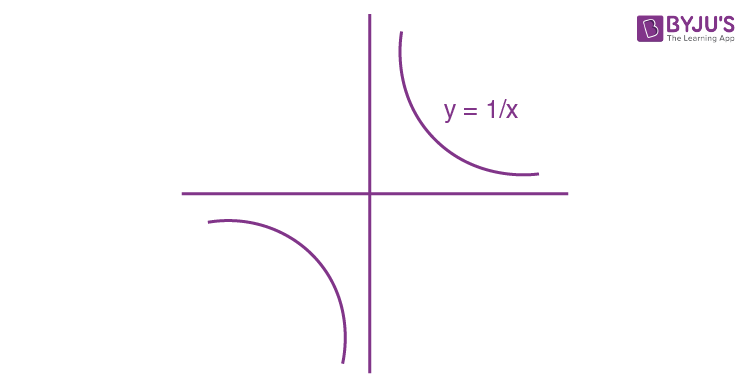Eccentricity of rectangular hyperbola

Also, xy = c

e.g. xy = 1, y = 1/x

### Tangent of Rectangular hyperbola

The tangent of a rectangular hyperbola is a line that touches a point on the rectangular hyperbola’s curve. The equation and slope form of a rectangular hyperbola’s tangent is given as:

#### Equation of tangent

The y = mx + c write hyperbola x2/a2 – y2/b2 = 1 will be tangent if c2 = a2/m2 – b2.

#### Slope form of tangent

y = mx ± √(a2m2 – b2)

#### Secant

Secant will cut ellipse at 2 distinct points

⇒ c2 > a2 m2 – b2

#### Neither Secant Nor Tangent

For line to be neither secant nor tangent, quadratic equation will give imaginary solution.

⇒ c2 < a2 m2 – b2

Equation of tangent to hyperbola x2 /a2 – y2/b2 = 1 at point (x1 ,y1) is

(xx1)/a2 = (yy1)/b1 = 1

Parametric form of tangent:

(x secθ) /a − (y tanθ)/b = 1

Point of contact and examples on tangent

Compare:

y = mx + c

(xx1)/a2 – (yy1)/b2 = 1 – mx + y = c

x1 = (-a2c)/m;

y1 = -b2/c

(x1,y1) = [(-a2m)/c, -b2/c]

### Solved Examples on Hyperbola

Example 5: Find the equation of tangent to hyperbola x2/9−y2=1 whose slope is 5

Solution:

Slope of tangent m = 5, a2 = 9, b2 = 1

Equation of tangent in slope form is

y = mx ± √(a2m2 – b2 )

y = 5x ± √(9.52 – 1)

y = 5x ± 4√14

[Note: For ellipse, director circle is x2 + y2 = a2 + b2, x2/a2 + y2/b2 = 1]

Normal:

Equation of normal of x2/a2 – y2/b2 = 1 at (x1 ,y1)

a2x/x1 + b2y/y1 = a2 + b2

Example 6: Find normal at the point (6, 3) to hyperbola x2/18 − y2/9 = 1

Solution:

Equation of Normal at point (x1, y1) is a2 = 18, b2 = 9

a2x/x1 + b2y/y1 = a2 + b2

Equation of Normal at point (6, 3) is

18x/6 + 9y/3 = 18 + 9

x + y = 9

Example 7: Find equation of chord of Contact of point (2, 3) to hyperbola x2/16 − y2/9 = 1

Solution:

Equation of chord of Contact is T = 0

i.e. (xx1)/a2 – (yy1)/b2 – 1 = 0

Or, 2x/16 – 3y/9 = 1

Or, x/8 – y/3 = 1

Equation of chord when mid-point is given

T = (xx1)/a2 – (yy1)/b2 – 1 = x12/a2 – y12/b2 − 1.

Example 9: Find the equation of chord of hyperbola x2/9 – y2/4 = 1 whose midpoint is (5, 1).

Solution:

We know, equation of chord of the hyperbola whose mid points is (x1, y1)

T = (xx1)/a2 – (yy1)/b2 – 1 = x12/a2 – y12/b2 − 1

Given, midpoint is (5, 1).

=>5x/9 – y/4 – 1= 25/9 – ¼ – 1

=>5x/9 – y/4 = 91/36

### Ellipse and Hyperbola Important JEE Main Questions### Ellipse and Hyperbola – Important Topics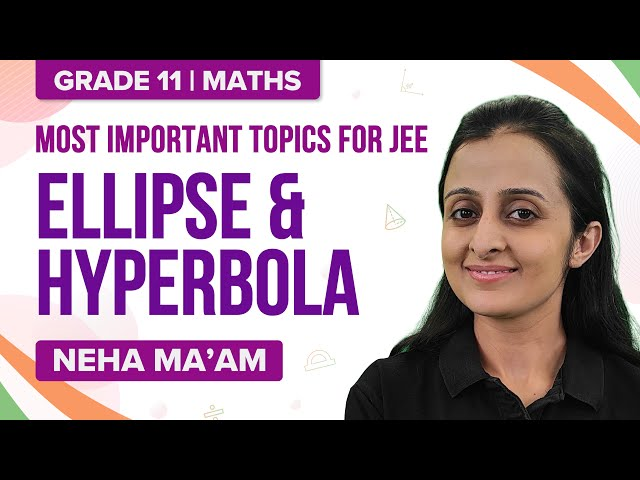### Ellipse and Hyperbola – Important Questions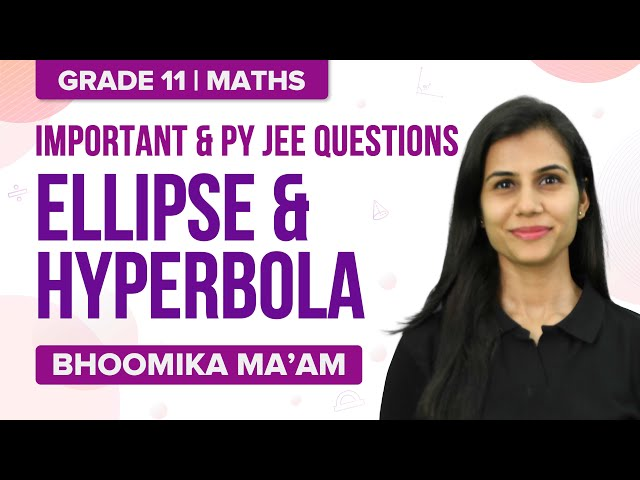### What is a Hyperbola?

Hyperbola is the locus of a point moving in a plane such that the ratio of its distance from a fixed point to that from a fixed line is a constant greater than 1.

### What is the eccentricity of Hyperbola?

The eccentricity of Hyperbola is greater than 1.

### What is the formula of eccentricity of Hyperbola?

The eccentricity is given by e = √(1 + (b2/a2)).

### Give the standard equation of Hyperbola.

The standard equation of a hyperbola is (x2/a2) – (y2/b2) = 1.